Ed Pegg Jr

## Ripple EffectNBCDFPDF

### B E Y O N D S U D O K U

Sudoku, today’s most well-known grid-solving puzzle, was originally invented by Howard Garns in 1978. NIKOLI Co., Ltd., a magazine publisher, introduced the puzzle in Japan during the 1980s. Almost 30 years later, its popularity suddenly exploded. Bookstores now carry dozens of Sudoku books.

Eventually, other puzzle types will explode in popularity. This new column presents obscure logic puzzles of various sorts and challenges the readers to solve the puzzles in two ways: by hand and with Mathematica. For the latter, solvers are invited to send their code to edp@wolfram.com. The person submitting the most elegant solution will receive a prize.

### Instructions

Ripple Effect was first published in Puzzle Communication Nikoli in May 1998 . In these puzzles, a grid is divided into polyomino-shaped rooms.

1. Each room contains consecutive numbers starting from 1. Thus, a domino will always have 1 and 2. A triomino will always have 1, 2, and 3. And so on.
2. If a number is duplicated in a row or a column, the space between the duplicated numbers must be equal to or larger than the value of the number. Thus, there will always be at least one digit between 1s, at least two digits between 2s, and so on.

Here is a solved example puzzle. The first grid is the puzzle itself. In the second, some preliminary logic has been done. To start, all single squares need a 1. Ones cannot be adjacent, which forces a few more placements. The square marked “A” cannot be a 2, since the domino above it could not be filled. With further logic, the entire puzzle is solved.

### Example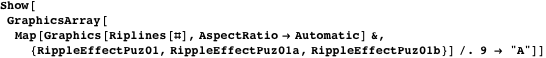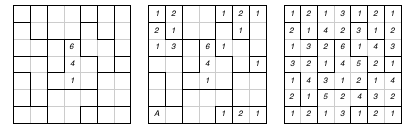### Puzzles

Here are two warm-up puzzles to solve, both from the Puzzle Cyclopedia . See the Puzzle Source section for the puzzle entry code and the Code section for the graphics.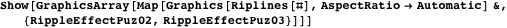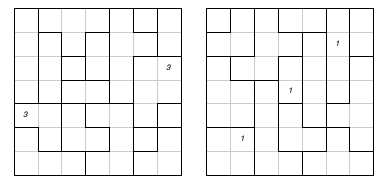Here are some intermediate puzzles from Puzzle Communication Nikoli .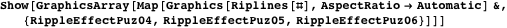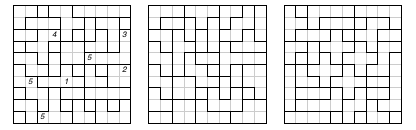Finally, here is a challenge puzzle from Puzzle Communication Nikoli .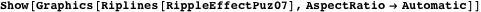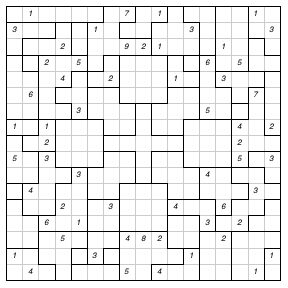Do let us know what you think of this new column. Send your comments to edp@wolfram.com.

### Puzzle Source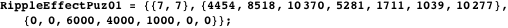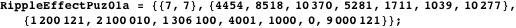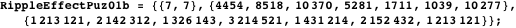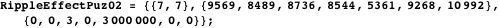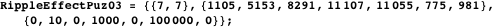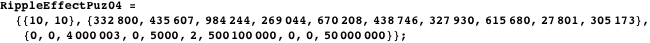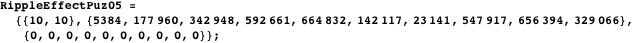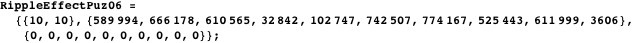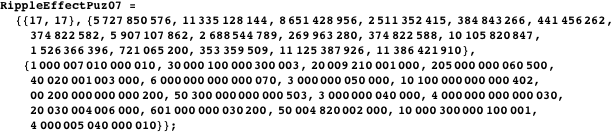### Code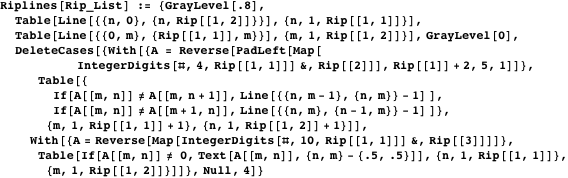### References

  Puzzle Communication Nikoli, 73, ISBN 4890724060, Tokyo, Japan: NIKOLI Co., Ltd., May 1998. www.nikoli.co.jp/howtoget-e.htm.  Puzzle Cyclopedia, ISBN 489072060, Tokyo, Japan: NIKOLI Co., Ltd., 2004. www.nikoli.co.jp/howtoget-e.htm.  Puzzle Communication Nikoli, 107, ISBN 4890726071, Tokyo, Japan: NIKOLI Co., Ltd., Summer Issue. www.nikoli.co.jp/howtoget-e.htm. E. Pegg Jr, “Ripple Effect,” The Mathematica Journal, 2012. dx.doi.org/10.3888/tmj.10.2-3.